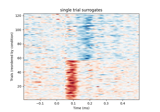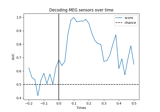# mne.decoding.EMS#

class mne.decoding.EMS[source]#

Transformer to compute event-matched spatial filters.

This version of EMS  operates on the entire time course. No time window needs to be specified. The result is a spatial filter at each time point and a corresponding time course. Intuitively, the result gives the similarity between the filter at each time point and the data vector (sensors) at that time point.

Note

EMS only works for binary classification.

References

Attributes:
filters_`ndarray`, shape (n_channels, n_times)

The set of spatial filters.

classes_`ndarray`, shape (n_classes,)

The target classes.

Methods

 `fit`(X, y) Fit the spatial filters. `fit_transform`(X[, y]) Fit to data, then transform it. `get_params`([deep]) Get the estimator params. `set_params`(**params) Set parameters (mimics sklearn API). Transform the data by the spatial filters.
fit(X, y)[source]#

Fit the spatial filters.

Parameters:
X`array`, shape (n_epochs, n_channels, n_times)

The training data.

y`array` of `int`, shape (n_epochs)

The target classes.

Returns:
selfinstance of `EMS`

Returns self.

Examples using `fit`:Compute effect-matched-spatial filtering (EMS)

Compute effect-matched-spatial filtering (EMS)
fit_transform(X, y=None, **fit_params)[source]#

Fit to data, then transform it.

Fits transformer to `X` and `y` with optional parameters `fit_params`, and returns a transformed version of `X`.

Parameters:
X`array`, shape (n_samples, n_features)

Training set.

y`array`, shape (n_samples,)

Target values or class labels.

**fit_params`dict`

Additional fitting parameters passed to the `fit` method..

Returns:
X_new`array`, shape (n_samples, n_features_new)

Transformed array.

get_params(deep=True)[source]#

Get the estimator params.

Parameters:
deep`bool`

Deep.

set_params(**params)[source]#

Set parameters (mimics sklearn API).

Parameters:
**params`dict`

Extra parameters.

Returns:
instobject

The instance.

transform(X)[source]#

Transform the data by the spatial filters.

Parameters:
X`array`, shape (n_epochs, n_channels, n_times)

The input data.

Returns:
X`array`, shape (n_epochs, n_times)

The input data transformed by the spatial filters.

Examples using `transform`:Compute effect-matched-spatial filtering (EMS)

Compute effect-matched-spatial filtering (EMS)

## Examples using `mne.decoding.EMS`#Decoding (MVPA)

Decoding (MVPA)Compute effect-matched-spatial filtering (EMS)

Compute effect-matched-spatial filtering (EMS)